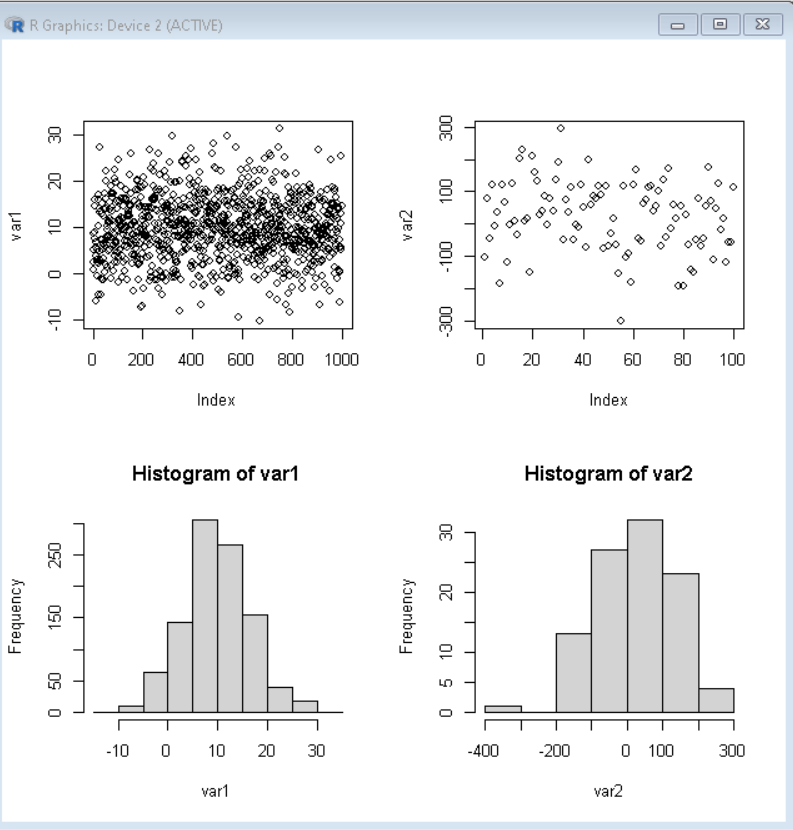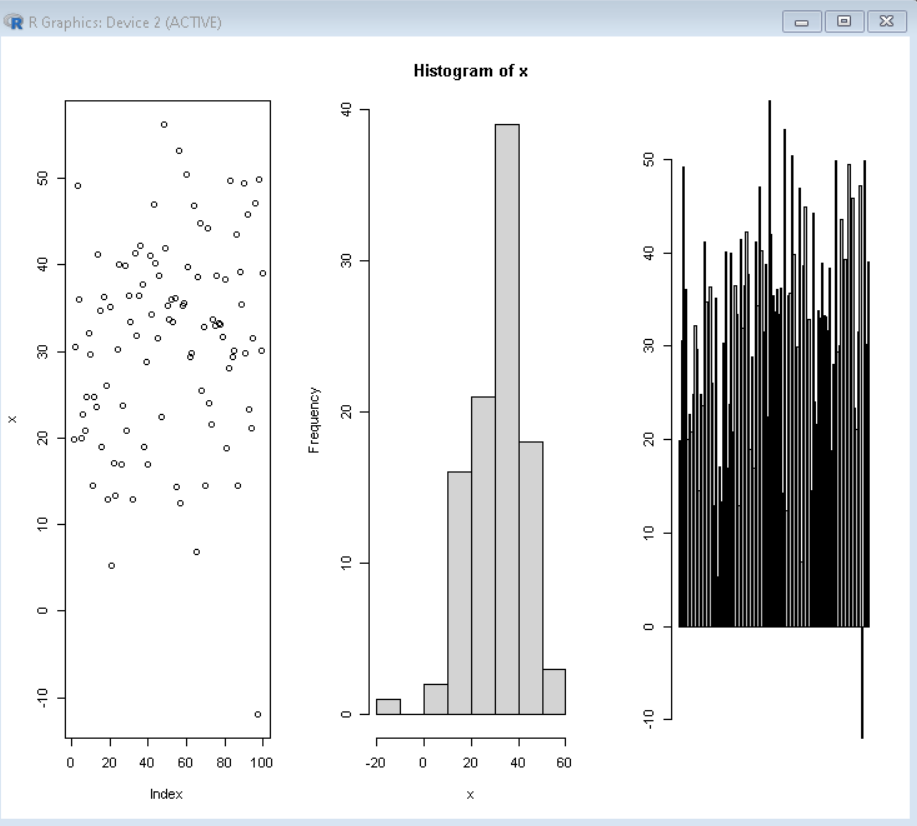# R ggplot2 – Multi Panel Plots

• Last Updated : 30 Jan, 2022

In this article, we are going to see how to plot Multi Panel Plots using ggplot2 in R Programming language.

Plots are one of the most important aspects of data visualization. They help us to quickly identify trends and relationships in the raw data. But sometimes one plot is not enough to derive the required relationship, thus, we need multiple plots simultaneously. Multi-panel plots refer to the plot creation of multiple graphs together in a single plot. This helps us in giving different visualization of the same data as well as visualization of a few different datasets in a single plot.

To create Multi Panel Plots in the R Language, we first divide the plot frame into the desired number of rows and columns and then fill those with desired plots. To divide the plot frame into the desired number of rows and columns, we use the par() function of the R Language. The par() function can be used to set or query graphical parameters. The mfrow argument of par() function takes a vector as a value that contains the number of rows and number of columns and returns a blank frame divided into those numbers of rows and columns.

Syntax: par( mfrow= c( col, row ) )

Parameters:

• col: determines the number of columns in which frame is to be divided.
• row: determines the number of rows in which frame is to be divided.

### Example1: Four plots in a 2X2 grid.

Here we are going to create a vector and then divide the frame into 2×2 grid and then plot multi-panel Plots.

## R

 `# Create Sample data ` `var1 <- ``rnorm``(1000,10,7) ` `var2 <- ``rnorm``(100,30,98) ` ` `  `# divide frame in 2X2 grid ` `par``( mfrow= ``c``(2,2) ) ` ` `  `# draw 4 plots ` `plot``( var1 ) ` `plot``( var2 ) ` `hist``( var1 ) ` `hist``( var2 )`

Output:## R

 `# Create Sample data ` `x <- ``rnorm``(100,30,13) ` ` `  `# divide frame in 1X3 ` `par``( mfrow= ``c``(1,3) ) ` ` `  `# draw 3 plots  ` `plot``( x ) ` `hist``( x ) ` `barplot``( x )`

Output:My Personal Notes arrow_drop_up
Recommended Articles
Page :